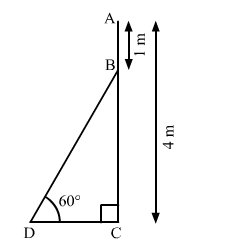# An electrician has to repair an electric fault on a pole of height 4 metres. He needs to reach a point 1 metre below the top of the pole to undertake the repair work.`
Question:

An electrician has to repair an electric fault on a pole of height 4 metres. He needs to reach a point 1 metre below the top of the pole to undertake the repair work. What should be the length of the ladder that he should use, which when inclined at an angle of 60° to the horizontal would enable him to reach the required position? $[$ Use $\sqrt{3}=$1.73]

Solution:Let AC be the pole and BD be the ladder.

We have,

$\mathrm{AC}=4 \mathrm{~m}, \mathrm{AB}=1 \mathrm{~m}$ and $\angle \mathrm{BDC}=60^{\circ}$

And, $B C=A C-A B=4-1=3 \mathrm{~m}$

In $\Delta \mathrm{BDC}$,

$\sin 60^{\circ}=\frac{\mathrm{BC}}{\mathrm{BD}}$

$\Rightarrow \frac{\sqrt{3}}{2}=\frac{3}{\mathrm{BD}}$

$\Rightarrow \mathrm{BD}=\frac{3 \times 2}{\sqrt{3}}$

$\Rightarrow \mathrm{BD}=2 \sqrt{3}$

$\Rightarrow \mathrm{BD}=2 \times 1.73$

$\therefore \mathrm{BD}=3.46 \mathrm{~m}$

So, he should use 3.46 m long ladder to reach the required position.